By Long Luo

# 前言

，加上自己也曾做过 $2$ 次核磁共振，一直很好奇其原理，所以花了点时间弄懂了核磁共振成像原理。

# 一维傅里叶变换(1D Fourier Transform)

$c_{n}=\frac{\Delta\omega}{2\pi}\int_{-\infty}^{+\infty} f(t)e^{-jwt}dt$

$f(t)=\sum_{-\infty}^{+\infty}(\frac{\Delta\omega}{2\pi}\int_{-\infty}^{+\infty} f(t)e^{-jwt}dt)e^{j\omega t}=\int_{-\infty}^{+\infty}\frac{1}{2\pi}(\int_{-\infty}^{+\infty}f(t)e^{-j\omega t}dt)e^{j\omega t}d\omega$

$F(\omega)=\int_{-\infty}^{+\infty}f(t)e^{-j\omega t}dt$

$F(f)=\int_{-\infty}^{+\infty}f(t)e^{-j2\pi f t}dt$

$\textit{DFT(Discrete Fourier Transform)}$ 也就是 $t$$f$ 都是离散的傅里叶变换。

## 一维离散傅里叶变换

### 采样(Sampling)

$t \neq 0$$\delta(t)=0$$\int_{-\infty}^{+\infty}\delta(t)dt=1$

$\delta_s(t)=\sum_{n=-\infty}^{\infty}\delta(t-nT_s)$ ，其中 $T_s$ 为采样周期。

### 时域离散化计算

$X(\omega)=\int_{-\infty}^{+\infty}x(t)e^{-j\omega t}dt$

$X_s(\omega)=\int_{-\infty}^{\infty}(\sum_{n=-\infty}^{\infty}x(t)\delta(t-nT_s))e^{-j\omega t}dt$

$X_s(\omega)=\sum_{n=-\infty}^{\infty}\int_{-\infty}^{\infty}x(t)\delta(t-nT_s)e^{-jwt}dt$

### 频域离散化计算

$X(k\omega_0)=\frac{1}{T_0}\int_{0}^{T}(\sum_{n=0}^{N-1}x(t)\delta(t-nT_s))e^{-jkw_0t}dt$ ，其中 $\omega_0=\frac{2\pi}{T_0}$

$X(k\omega_0)=\frac{1}{T_0}\sum_{n=0}^{N-1}\int_{0}^{T}x(t)\delta(t-nT_s)e^{-jk\omega_0t}dt$

$X(k\omega_0)=\frac{1}{NT_s}\sum_{n=0}^{N-1}x(nTs)e^{-j\frac{2\pi}{NT_s}knT_s}=\frac{1}{NT_s}\sum_{n=0}^{N-1}x(nTs)e^{-j\frac{2\pi}{N}kn}$

$X[k]=\frac{1}{N}\sum_{n=0}^{N-1}x[n]e^{-j\frac{2\pi}{N}kn}$

## 一维傅里叶变换可视化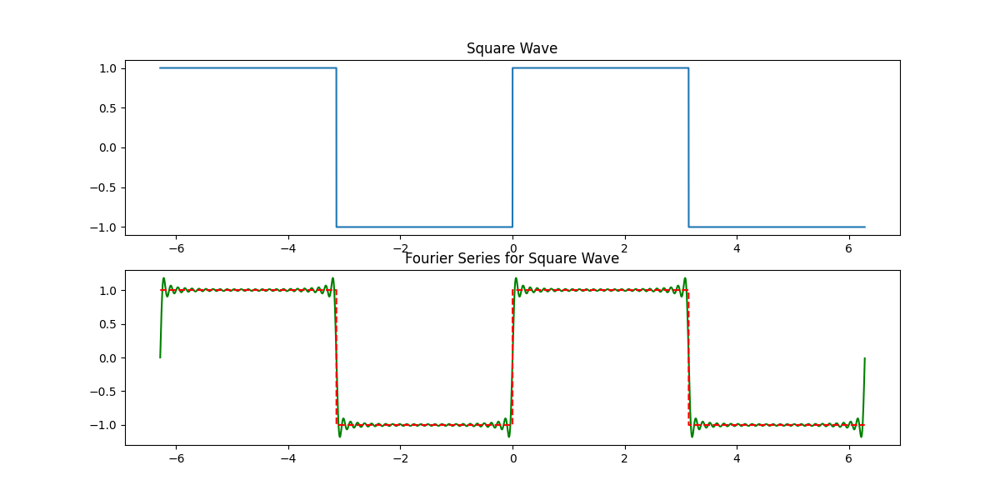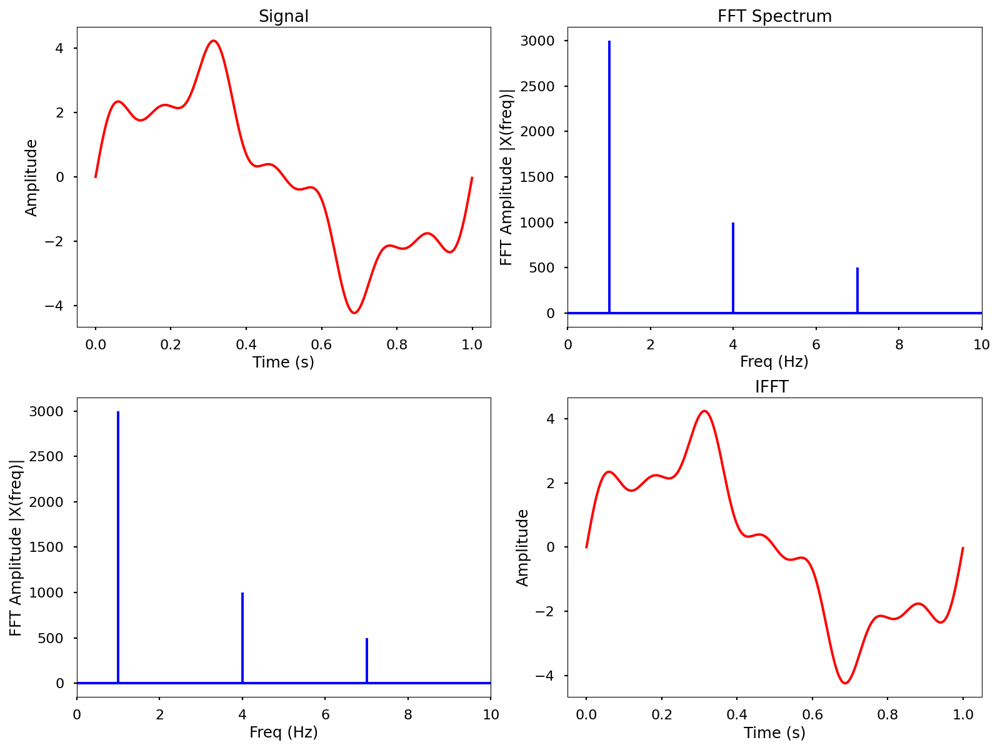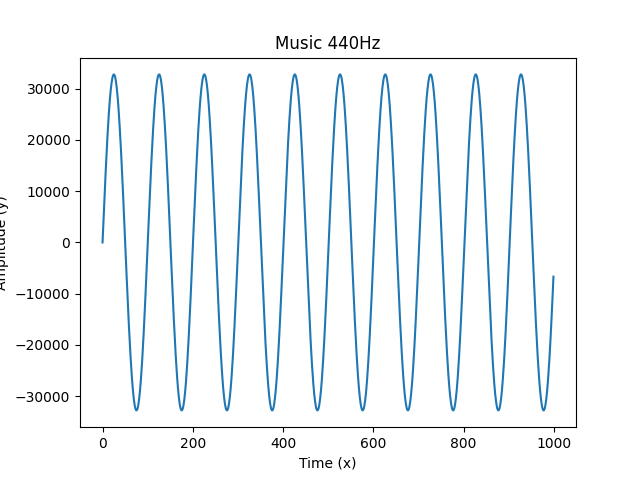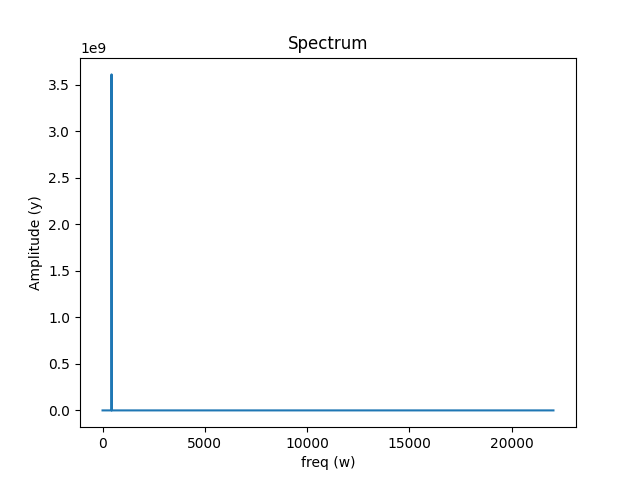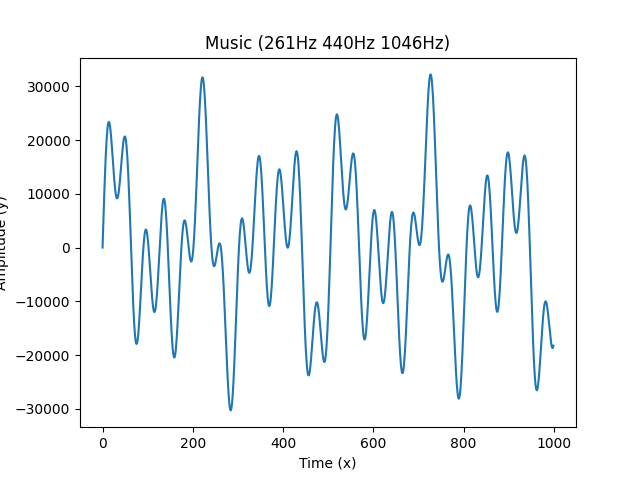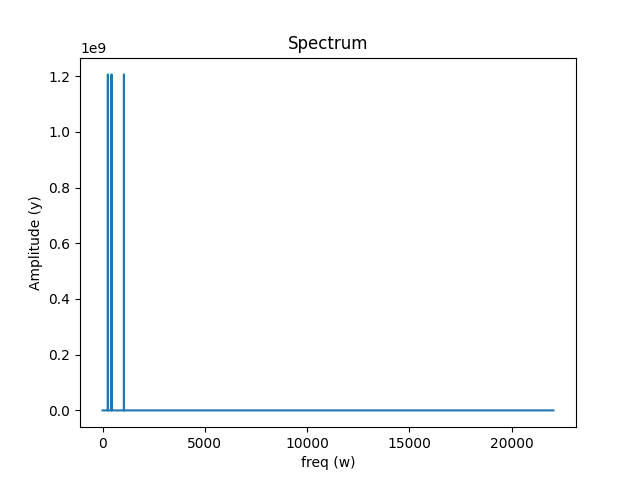# 二维连续傅里叶变换(2D Fourier Transforms)

## 二维连续傅里叶变换

$F(u,v) = \int_{-\infty }^{\infty}\int_{-\infty }^{\infty} f(x,y)e^{-j2\pi(ux+vy)}dxdy$

## 二维连续傅里叶逆变换

$f(x,y) = \frac{1}{4\pi^2} \int_{-\infty }^{\infty}\int_{-\infty }^{\infty} F(u,v)e^{j2\pi(ux+vy)}dudv$

# 二维离散傅里叶变换

## 二维离散傅里叶变换

$F(u,v)=\sum_{x=0}^{M-1} \sum_{y=0}^{N-1}f(x,y)e^{-j2\pi(\frac{ux}{M}+\frac{vy}{N})}$

## 二维离散傅里叶逆变换

$f(x,y)=\frac{1}{MN}\sum_{x=0}^{M-1} \sum_{y=0}^{N-1}F(u,v)e^{j2\pi(\frac{ux}{M}+\frac{vy}{N})}$

# 傅里叶变换特征参数

$F(u,v)=R(u,v)+jI(u,v)$

## 频谱/幅度谱

$|F(u,v)|=\sqrt{R^2(u,v)+I^2(u,v)}$

$P(u,v)=R^2(u,v)+I^2(u,v)$

$\psi (u,v)=arctan\frac{I(u,v)}{R(u,v)}$